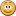## Alphanum: Javascript Natural Sorting Algorithm

Jan 16, 2008

Today I had a need for a natural sorting function in javascript. I've seen these functions before in other languages, so I thought to myself, no problem, I'll find one in a couple of minutes! How wrong I was!

First off, a little explanation. Natural sorting, or Lexicographical order, is sorting a list of strings which contain letters and numbers, in such a way that makes sense to humans rather than computers. Given a list of filenames, a computer would sort them this way:

`z1.doc, z10.doc, z17.doc, z2.doc, z23.doc, z3.doc`

While a human would sort it like so:

`z1.doc, z2.doc, z3.doc, z10.doc, z17.doc, z23.doc`

Obviously, the second list makes more sense, no? Anyway, I needed an algorithm that would sort any list of strings in that fashion.

Right off the bat, I found two promising candidates, but both failed quite spectacularly on my test array (which included bunches of numbers up to 1000). More searching only seemed to find blog posts which merely linked to these faulty examples.

So, giving up that angle, I decided the best way to get a natural sorting algorithm in javascript was to examine one from another language (preferably one which worked) and port it to javascript.

I ended up coming across David Koelle's Alphanum algorithm which worked like a charm. I took the Perl implementation and ported it to Javascript resulting in the function below:

```function alphanum(a, b) {
function chunkify(t) {
var tz = [], x = 0, y = -1, n = 0, i, j;

while (i = (j = t.charAt(x++)).charCodeAt(0)) {
var m = (i == 46 || (i >=48 && i <= 57));
if (m !== n) {
tz[++y] = "";
n = m;
}
tz[y] += j;
}
return tz;
}

var aa = chunkify(a);
var bb = chunkify(b);

for (x = 0; aa[x] && bb[x]; x++) {
if (aa[x] !== bb[x]) {
var c = Number(aa[x]), d = Number(bb[x]);
if (c == aa[x] && d == bb[x]) {
return c - d;
} else return (aa[x] > bb[x]) ? 1 : -1;
}
}
return aa.length - bb.length;
}```
Note that this code is case sensitive, so "A" will sort before "a". You can turn it into a case insensitive sort by lowercasing the incoming a and b test strings. Just change the chunkify lines to:
```  var aa = chunkify(a.toLowerCase());
var bb = chunkify(b.toLowerCase());```
Thanks to David K. for the algorithm! This saved me tons of time. I owe you one.An IE Issue Appears

After first posting this script, I came across an issue in IE which caused the script to fail. Don't worry! The lines of code above are the modified - and working - alphanum function. The problem occurred in the script as originally posted; specifically with the two lines:

```  a = chunkify(a);
b = chunkify(b);```
Note the variable names, where the assignment tries to place the result back into the original variable. Actually, all that's required for the bug to manifest is to assign any array to the incoming a and b variables. For some strange reason this would fail in IE6 and IE7 while sorting arrays with larger than 23 or 24 elements. In these cases, at seemingly random times during the sort, "undefined" would be passed back to a, causing the next reference to it to trigger an exception.

The following is example code (from a testcase prepared by TarquinWJ) which triggers the bug in IE. This sort will fail in IE6 and IE7.

```function customsort(a, b) {
a = ['a']; // assign an array to a
b = ['b']; // assign an array to b

// IE will bail out when either a or b is undefined
return a.length - b.length;
}
var arr = [
'a','b','c','d','e','f','g','h','i','j','k','l','m',
'n','o','p','q','r','s','t','u','v','w','x'
];
arr.sort(customsort);```
To solve this, all it took was to assign the result to new variables (aa and bb) and change all further references to a and b to the new set. Definitely a JS bug in IE, and as it involves the creation and destruction of many arrays within a short time, it is likely some kind of memory issue.

Thanks very much for TarquinWJ for helping isolate this bug.

A Faster Prototype Version

For those who value speed over flexibility, I also made this function into an Array method. Because it breaks up all the array strings first, then does the actual sorting, then joins all the sub-arrays back into strings, it is a great deal faster than the version above. That version breaks up two strings for each sort iteration rather than only once for each array element.

It's harder to modify this method to sort multi-dimensional arrays, or arrays of objects with string properties, but the speed gain is hard to ignore. As an added bonus, you can specify case sensitivity on a per-call basis with the caseInsensitive argument (true|false). If you're just going to sort plain arrays of strings, use this version.

```Array.prototype.alphanumSort = function(caseInsensitive) {
for (var z = 0, t; t = this[z]; z++) {
this[z] = [];
var x = 0, y = -1, n = 0, i, j;

while (i = (j = t.charAt(x++)).charCodeAt(0)) {
var m = (i == 46 || (i >=48 && i <= 57));
if (m !== n) {
this[z][++y] = "";
n = m;
}
this[z][y] += j;
}
}

this.sort(function(a, b) {
for (var x = 0, aa, bb; (aa = a[x]) && (bb = b[x]); x++) {
if (caseInsensitive) {
aa = aa.toLowerCase();
bb = bb.toLowerCase();
}
if (aa !== bb) {
var c = Number(aa), d = Number(bb);
if (c == aa && d == bb) {
return c - d;
} else return (aa > bb) ? 1 : -1;
}
}
return a.length - b.length;
});

for (var z = 0; z < this.length; z++)
this[z] = this[z].join("");
}```